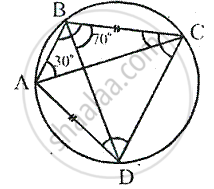Share

# In Cyclic Quadrilateral Abcd; Ad = Bc, ∠Bac = 30° and ∠Cbd = 70°; Find: (I) ∠Bcd (Ii) ∠Bca (Iii) ∠Abc (Iv) ∠Adc - Mathematics

Course

#### Question

In cyclic quadrilateral ABCD; AD = BC, ∠BAC = 30° and ∠CBD = 70°; find:
(i) ∠BCD (ii) ∠BCA (iii) ∠ABC (iv) ∠ADC

#### Solution∠BAC = 30° , ∠CBD = 70°

∠DAC = ∠CBD [Angles in the same segment]
⇒ ∠DAC = 70°     [ ∵ CBD = 70°]
⇒ ∠BAD = ∠BAC + ∠DAC = 30° + 70° = 100°
Since the sum of opposite angles of cyclic quadrilateral is supplementary
⇒ 100°+ ∠BCD = 180°         [From (1)]

⇒ ∠BCD = 180° -100° = 80°
Since , AD = BC ,∠ACD = ∠BDC    [Equal chords subtends equal angles]

But ∠ACB = ∠ADB     [angles in the same segment]

∴  ∠ACD + ∠ACB = ∠BDC + ∠ADB
⇒ ∠BCD = ∠ADC = 80°
But in ∆BCD,
∠CBD + ∠BCD + ∠BDC = 180 [angles oaf a triangle]
⇒ 70° + 80° + ∠BDC = 180°
⇒  150° + ∠BDC = 180°
∴  ∠BDC = 180° -150° = 30°

⇒ ∠ACD = 30° [∠ACD = ∠BDC ]

∴ ∠BCA = ∠BCD - ∠ACD = 80° - 30° = 50°

Since the sum of opposite angles of cyclic quadrilateral is supplementary
⇒ 80° + ABC = 180°
⇒ ∠ABC =180° - 80° = 100°

Is there an error in this question or solution?